Note: This is a working paper which will be expanded/updated frequently. All suggestions for improvement are welcome. The directory deleeuwpdx.net/pubfolders/above has a pdf version, the complete Rmd file with all code chunks, the bib file, and the R source code.

# 1 Introduction

In this paper we study the minimization of stress, defined as $\mathbf{stress}(X):=\frac14\sum_{i=1}^n\sum_{j=1}^n w_{ij}(\delta_{ij}-d_{ij}(X))^2$ over $$n\times p$$ configuration matrices $$X$$. Here $$W=\{w_{ij}\}$$ and $$\Delta=\{\delta_{ij}\}$$ are given symmetric, hollow, and non-negative matricesof, respectively, weights and dissimilarities. The $$d_{ij}(X)$$ are Euclidean distances between the points with coordinates in the rows of $$X$$. Thus $d_{ij}^2(X):=(x_i-x_j)'(x_i-x_j)=(e_i-e_j)'XX'(e_i-e_j)=\mathbf{tr}\ X'A_{ij}X.$ Here $$e_i$$ and $$e_j$$ are unit vectors, with all elements equal to zero except for the single element $$i$$ or $$j$$, which is equal to one. Also $$A_{ij}:=(e_i-e_j)(e_i-e_j)'$$.

The problem of minimizing stress is well-studied (see, for example, Borg and Groenen (2005)). In this paper we analyze the problem of minimizing stress over all configurations $$X$$ for which $$d_{ij}(X)\geq\alpha_{ij}$$ for some or all pairs $$i,j$$, where the $$\alpha_{ij}$$ are positive bounds. As a special case we have MDS from above, where we require $$d_{ij}(X)\geq\delta_{ij}$$ for all $$i,j$$.

These optimization problems are non-standard in various ways. The constraints $$d_{ij}(X)\geq\alpha_{ij}$$ define a reverse convex set (Meyer (1970)) in configuration space, and in addition stress is not convex. We can, however, use basic smacof theory (De Leeuw (1977)) to majorize stress by a convex quadratic and to majorize the constraints by linear functions. Majorization can be used to define a simple iterative algorithm. In each iteration we majorize stress and the constraints, and then minimize the convex quadratic majorizer over configurations satisfying the majorized linear constraints using quadratic programming. Compute a new majorization at the minimizer of the majorized problem, and so on. These are the two steps in the majorization or MM approach to optimization (De Leeuw (1994), Lange (2016)).

# 2 Some Majorization Theory

Suppose the problem we are interested in is to minimize a real-valued $$f_0$$ over all $$x\in\mathbb{R}^n$$ that satisfy $$f_j(x)\leq 0$$ for all $$j=1,\cdots,m$$. We call this problem $$\mathcal{P}$$.

Now suppose $$g_j:\mathbb{R}^n\otimes\mathbb{R}^n\rightarrow\mathbb{R}$$ majorizes $$f_j$$, i.e. \begin{align*} f_j(x)&\leq g_j(x,y)\text{ for all }x,y\in\mathbb{R}^n,\\ f_j(y)&=g_j(y,y)\text{ for all }y\in\mathbb{R}^n. \end{align*}

To simplify matters we suppose all $$f_j$$ and $$g_j$$ are continuously differentiable, and all $$g_j$$ are convex in their first argument. Define problem $$\mathcal{P}_k$$ as the problem of minimizing $$g_0(x,x^{(k-1)})$$ over the $$x\in\mathbb{R}^n$$ that satisfy $$g_j(x,x^{(k-1)})\leq 0$$. Define a sequence $$x^{(k)}$$ with $$x^{(0)}$$ feasible for $$\mathcal{P}$$, and with $$x^{(k)}$$ for $$k\geq 1$$ a solution of $$\mathcal{P}_k$$.

Theorem 1: [Convergence]

1. $$x^{(k)}$$ is feasible for $$\mathcal{P}$$ for all $$k\geq 1$$.
2. $$f_0(x^{(k)})\leq f_0(x^{(k-1)})$$ for all $$k\geq 1$$.

Proof: For the first part $$f_j(x^{(k)})\leq g_j(x^{(k)},x^{(k-1)})$$ by majorization, and $$g_j(x^{(k)},x^{(k-1)})\leq 0$$ by feasibility for $$\mathcal{P}_k$$. For the second part $$f_0(x^{(k)})\leq g_0(x^{(k)},x^{(k-1)})$$ by majorization. Because $$x^{(k)}$$ solves $$\mathcal{P}_k$$ we have $$g_0(x^{(k)},x^{(k-1)})\leq g_0(x^{(k-1)},x^{(k-1)})$$ if $$x^{(k-1)}$$ is feasible for $$\mathcal{P}_k$$, i.e. if $$g_j(x^{(k-1)},x^{(k-1)})\leq 0$$. But $$g_j(x^{(k-1)},x^{(k-1)})=f_j(x^{(k-1)})\leq 0$$ by the first part of the theorem. QED

# 3 Fixed Points

By theorem 1 we have a sequence of points with decreasing function values that are all feasible for $$\mathcal{P}$$. We can say a bit more about accumulation points of this sequence.

Define $$\mathcal{P}(y)$$ as the convex problem of minimizing $$g_0(x,y)$$ over $$x\in\mathbb{R}^n$$ and $$g_j(x,y)\leq 0$$.

Theorem 2: [Necessary]

If $$x$$ solves $$\mathcal{P}(x)$$ then $$x$$ satisfies the first order necessary conditions for a local minimum of $$\mathcal{P}$$.

Proof: The necessary and sufficient conditions for $$x$$ to be a minimum of $$\mathcal{P}(y)$$ are that there exists $$\lambda\geq 0$$ such that $\mathcal{D}_1g_0(x,y)+\sum_{j=1}^m\lambda_j\mathcal{D}_1g_j(x,y)=0,$ with in addition $$g_j(x,y)\leq 0$$ and $$\lambda_jg_j(x,y)=0$$.

The first order necessary conditions for $$x$$ to be a local minimum of $$\mathcal{P}$$ are that there exists $$\lambda\geq 0$$ such that $\mathcal{D}f_0(x)+\sum_{j=1}^m\lambda_j\mathcal{D}f_j(x)=0,$ with in addition $$f_j(x)\leq 0$$ and $$\lambda_jf_j(x)=0$$.

But if the $$f_j$$ and $$g_j$$ are differentiable, we know from majorization theory (De Leeuw (2016), Lange (2016)) that $$\mathcal{D}f_j(x)=\mathcal{D}_1g_j(x,x)$$ for all $$x$$.

QED

It follows that if we define $$\mathcal{F}(y)$$ as the solution of $$\mathcal{P}(y)$$ then fixed points of $$\mathcal{F}$$ are local minimizers of $$\mathcal{P}$$. Thus if $$x^{(k)}$$ converges to a fixed point of $$\mathcal{F}$$, it converges to a local minimizer of $$\mathcal{P}$$, and $$f_0(x^{(k)})$$ converges to a local minimum.

# 4 MDS with Lower Bounds

We have see in the companion paper (De Leeuw (2017)) that we can write $\mathbf{stress}(x)=1-\rho(x)+\frac12 x'x,$ where $\rho(x):=\sum_{k=1}^K w_k\delta_k d_k(x),$ with distances $$d_k(x):=\sqrt{x'A_kx}$$, and the $$A_k$$ positive semi-definite matrices that add up to the identity. Define $B(x):=\sum_{k=1}^K w_k\frac{\delta_k}{d_k(x)}A_k,$ and define the Guttman transform if $$x$$ as $$\Gamma(x):=B(x)x$$, then for all $$x$$ $\mathbf{stress}(x)=1-x'\Gamma(x)+\frac12 x'x=(1-\frac12\Gamma(x)'\Gamma(x))+\frac12(x-\Gamma(x))'(x-\Gamma(x)),$ and for all $$x,y$$ we have the majorization $\mathbf{stress}(x)\leq 1-x'\Gamma(y)+\frac12 x'x=(1-\frac12\Gamma(y)'\Gamma(y))+\frac12(x-\Gamma(y)'(x-\Gamma(y)),$ The constraints are $$\sqrt{x'A_kx}\geq\alpha_k$$ or $$\alpha_k-\sqrt{x'A_kx}\leq 0$$. The linearized constraints are $\alpha_k-\frac{1}{\sqrt{y'A_ky}}x'A_ky\leq 0$ Thus the quadratic programming problem we have to solve in each smacof iteration is minimization of $$1-x'\Gamma(x^{(k-1)})+\frac12 x'x$$ over $$x$$ satisfying $x'A_kx^{(k-1)}\geq\alpha_kd_k(x^{(k-1)}).$ We use the qp function from the lsei package (Wang, Lawson, and Hanson (2015)).

# 5 Examples

## 5.1 Equidistances

Our first example has $$n=10$$ with all $$\delta_{ij}$$ equal. The optimal smacof solution in two dimensions needs 131 iterations to arrive at stress 0.1098799783. We reanalyze the data with the constraint that the distance between the first point and all nine others is at least one. The algorithm incorporating these bounds, implemented in the function smacofAbove, uses 157 iterations and finds stress 0.1340105192. The two configurations are in figure 1.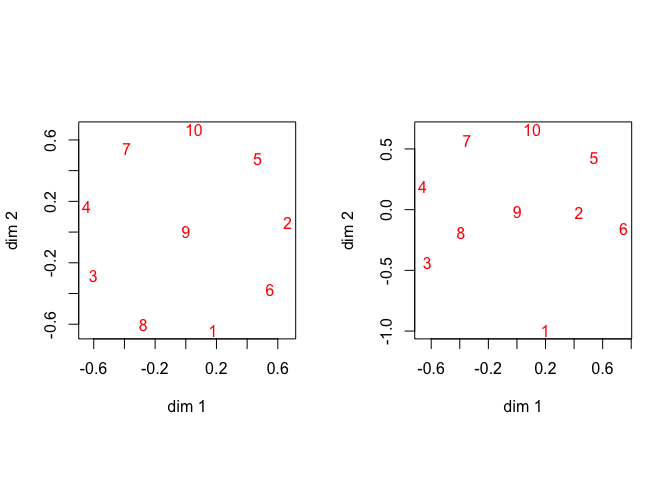Figure 1: Equidistance Data, Regular Left, Lower Bounds Right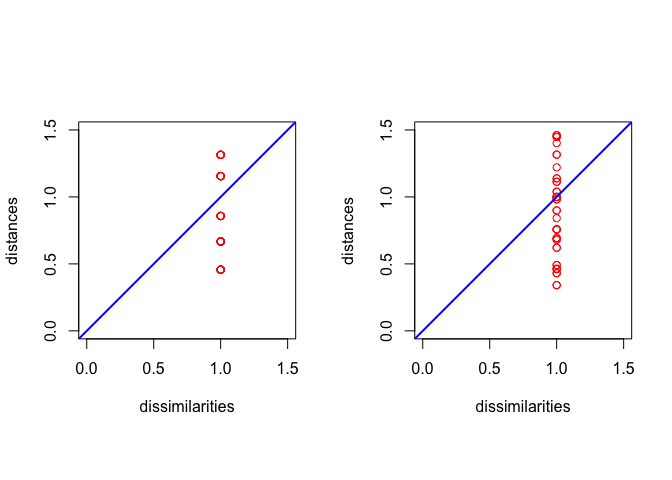Figure 2: Equidistance Data, Regular Left, Lower Bounds Right

## 5.2 Dutch Political Parties 1967

As the next illustration we use data from De Gruijter (1967), with average dissimilarity judgments between Dutch political parties in 1967. The data are

##       KVP PvdA  VVD  ARP  CHU  CPN  PSP   BP
## PvdA 5.63
## VVD  5.27 6.72
## ARP  4.60 5.64 5.46
## CHU  4.80 6.22 4.97 3.20
## CPN  7.54 5.12 8.13 7.84 7.80
## PSP  6.73 4.59 7.55 6.73 7.08 4.08
## BP   7.18 7.22 6.90 7.28 6.96 6.34 6.88
## D66  6.17 5.47 4.67 6.13 6.04 7.42 6.36 7.36

The optimal smacof solution in two dimensions needs 319 iterations to arrive at stress 0.044603386. Approximation from above, where we require that all distances are at least as large as the corresponding dissimilarties, uses 30 iterations and finds stress 0.2801306914. At the solution there are 15 active constraints, but as the Shepard plots in figure 4 show, the active constraints now do not correspond with the smallest dissimilarities, although they do correspond to the smallest distances.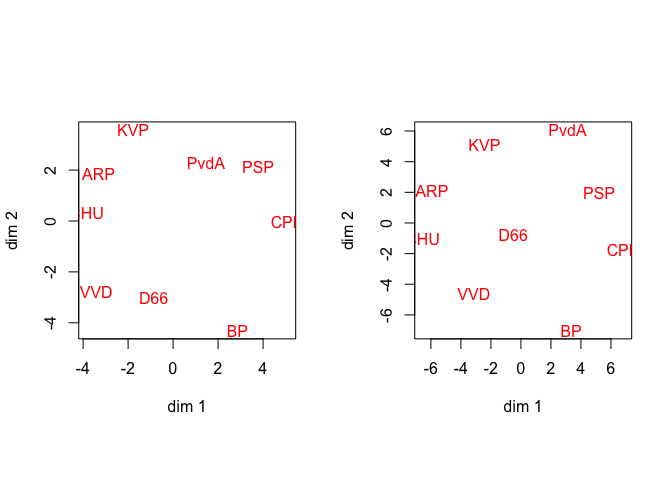Figure 3: De Gruijter Data, Regular Left, From Above Right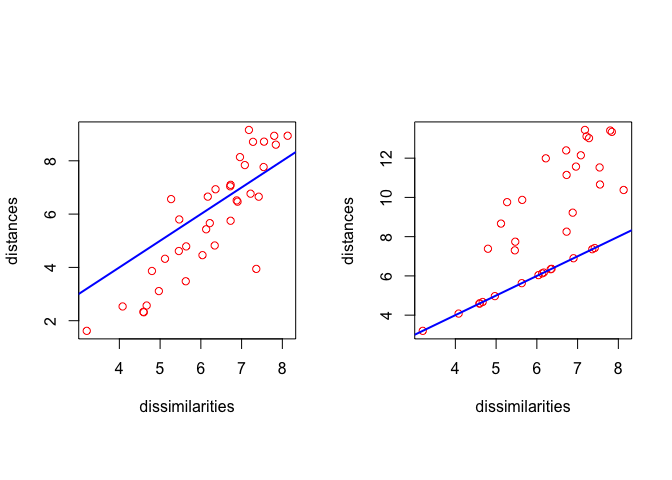Figure 4: De Gruijter Data, Regular Left, From Above Right

In the next analysis we require that all distances are larger than or equal to 3.2, the minimum dissimilarity. In order words, the smallest distances must be larger than or equal to the minimum dissimilarity.

The optimal solution under these constraints in two dimensions needs 128 iterations to arrive at stress 0.0509159458.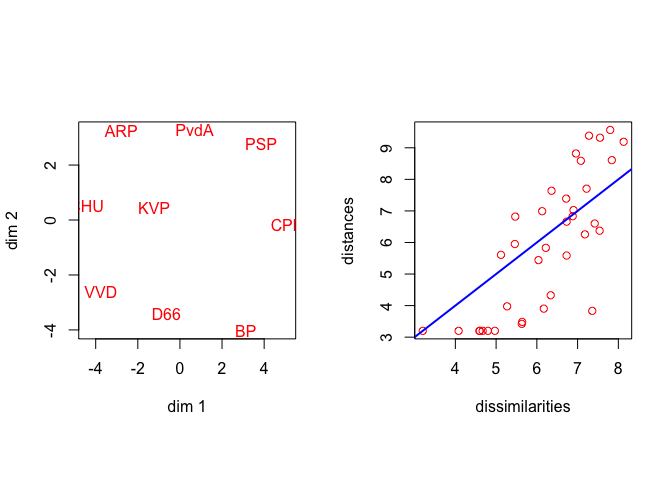Figure 5: De Gruijter Data, Distances Bounded by Minimum Dissimilarity

And finally an analysis in which we require that distances between ARP, CHU, KVP (the Christian Democrat parties) are larger than 5, and the distances between PvdA, PSP, CPN (the leftist parties) are also larger than 5. This forces them apart.

The optimal solution under these constraints in two dimensions needs 167 iterations to arrive at stress 0.0807378807. The six constrained distances are 5, 5, 5, 7.8645711944, 5.0000000003, 5.0000000001, so the Christian Democrats, for example, are on an equilateral triangle with side 5.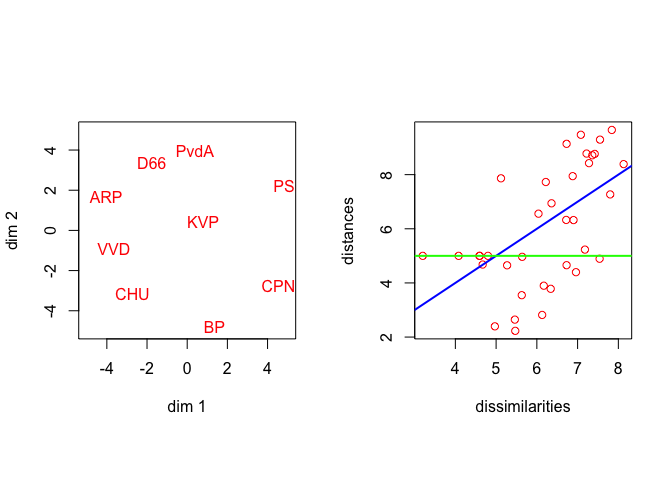Figure 6: De Gruijter Data, Imposing Large Distances

# 6 Discussion

The obvious next step is to combine the algorithms of De Leeuw (2017) and this paper to implement both upper and lower bounds on the distances. Of course for that case we have to be careful that a solution actually exists.

Another next step is to see in how far these bounding algorithms are useful for both metric and non-metric unfolding.

# 7 Appendix: Code

## 7.1 above.R

suppressPackageStartupMessages (library (mgcv, quietly = TRUE))
suppressPackageStartupMessages (library (lsei, quietly = TRUE))
source("auxilary.R")
source("mdsUtils.R")
source("smacof.R")
source("smacofAbove.R")

## 7.2 auxilary.R

mprint <- function (x,
d = 6,
w = 8,
f = "") {
print (noquote (formatC (
x,
di = d,
wi = w,
fo = "f",
flag = f
)))
}

directSum <- function (x) {
m <- length (x)
nr <- sum (sapply (x, nrow))
nc <- sum (sapply (x, ncol))
z <- matrix (0, nr, nc)
kr <- 0
kc <- 0
for (i in 1:m) {
ir <- nrow (x[[i]])
ic <- ncol (x[[i]])
z[kr + (1:ir), kc + (1:ic)] <- x[[i]]
kr <- kr + ir
kc <- kc + ic
}
return (z)
}

repList <- function(x, n) {
z <- list()
for (i in 1:n)
z <- c(z, list(x))
return(z)
}

shapeMe <- function (x) {
m <- length (x)
n <- (1 + sqrt (1 + 8 * m)) / 2
d <- matrix (0, n, n)
k <- 1
for (i in 2:n) {
for (j in 1:(i - 1)) {
d[i, j] <- d[j, i] <- x[k]
k <- k + 1
}
}
return (d)
}

symmetricFromTriangle <- function (x, lower = TRUE, diagonal = TRUE) {
k <- length (x)
if (diagonal)
n <- (sqrt (1 + 8 * k) - 1) / 2
else
n <- (sqrt (1 + 8 * k) + 1) / 2
if (n != as.integer (n))
stop ("input error")
nn <- 1:n
if (diagonal && lower)
m <- outer (nn, nn, ">=")
if (diagonal && (!lower))
m <- outer (nn, nn, "<=")
if ((!diagonal) && lower)
m <- outer (nn, nn, ">")
if ((!diagonal) && (!lower))
m <- outer (nn, nn, "<")
b <- matrix (0, n, n)
b[m] <- x
b <- b + t(b)
if (diagonal)
diag (b) <- diag(b) / 2
return (b)
}

triangleFromSymmetric <- function (x, lower = TRUE, diagonal = TRUE) {
n <- ncol (x)
nn <- 1:n
if (diagonal && lower)
m <- outer (nn, nn, ">=")
if (diagonal && (!lower))
m <- outer (nn, nn, "<=")
if ((!diagonal) && lower)
m <- outer (nn, nn, ">")
if ((!diagonal) && (!lower))
m <- outer (nn, nn, "<")
return (x[m])
}

## 7.3 mdsUtils.R

library (mgcv)

torgerson <- function (delta, p = 2) {
z <- slanczos(-doubleCenter((delta ^ 2) / 2), p)
w <- matrix (0, p, p)
v <- pmax(z$values, 0) diag (w) <- sqrt (v) return(z$vectors %*% w)
}

basisPrep <- function (n, p, w) {
m <- n * (n - 1) / 2
v <- -symmetricFromTriangle (w, diagonal = FALSE)
diag (v) <- -rowSums(v)
ev <- eigen (v)
eval <- ev$values[1:(n - 1)] evec <- ev$vectors[, 1:(n - 1)]
z <- evec %*% diag (1 / sqrt (eval))
a <- array (0, c(n - 1, n - 1, m))
k <- 1
for (j in 1:(n-1)) {
for (i in (j+1):n) {
dif <- z[i,] - z[j,]
a [, , k] <- outer (dif, dif)
k <- k + 1
}
}
return (list (z = z, a = a))
}

center <- function (x) {
return (apply (x, 2, function (z) z - mean (z)))
}

doubleCenter <- function (x) {
n <- nrow (x)
j <- diag(n) - (1 / n)
return (j %*% x %*% j)
}

squareDist <- function (x) {
d <- diag (x)
return (outer (d, d, "+") - 2 * x)
}

## 7.4 smacof.R

smacof <-
function (delta,
p = 2,
xini = torgerson (symmetricFromTriangle (delta, diagonal = FALSE), p),
w = rep (1, length (delta)),
itmax = 1000,
eps = 1e-10,
verbose = FALSE) {
m <- length (delta)
n <- (1 + sqrt (1 + 8 * m)) / 2
r <- p * (n - 1)
h <- basisPrep (n, p, w)
xold <- rep (0, r)
for (s in 1:p) {
k <- (s - 1) * (n - 1) + 1:(n - 1)
xold[k] <-
crossprod (h$z, xini[, s]) / diag (crossprod (h$z))
}
d <- rep (0, m)
itel <- 1
sold <- Inf
ssq <- sum (w * delta ^ 2)
repeat {
bmat <- matrix (0, n - 1, n - 1)
xx <- matrix (xold, n - 1, p)
for (k in 1:m) {
ak <- h$a[, , k] d[k] <- sqrt (sum (xx * (ak %*% xx))) bmat <- bmat + w[k] * (delta[k] / d[k]) * ak } xnew <- as.vector (bmat %*% xx) snew <- sum (w * (delta - d) ^ 2) / ssq if (verbose) cat( "Iteration: ", formatC (itel, width = 3, format = "d"), "sold: ", formatC ( sold, digits = 8, width = 12, format = "f" ), "snew: ", formatC ( snew, digits = 8, width = 12, format = "f" ), "\n" ) if ((itel == itmax) || ((sold - snew) < eps)) break xold <- drop (xnew) sold <- snew itel <- itel + 1 } xconf <- matrix (0, n, p) for (s in 1:p) { k <- (s - 1) * (n - 1) + 1:(n - 1) xconf[, s] <- h$z %*% xnew[k]
}
return (list (
delta = delta,
dist = d,
x = xconf,
itel = itel,
stress = snew
))
}

## 7.5 smacofAbove.R

smacofAbove <-
function (delta,
p = 2,
xini = torgerson (symmetricFromTriangle (delta, diagonal = FALSE), p),
w = rep (1, length (delta)),
bnd = rep (0, length (delta)),
itmax = 1000,
eps = 1e-10,
verbose = FALSE) {
m <- length (delta)
n <- (1 + sqrt (1 + 8 * m)) / 2
wnd <- which (bnd > 0)
l <- length (wnd)
xd <- as.vector (dist (xini))
lbd <- max (bnd[wnd] / xd[bnd])
xini <- lbd * xini
r <- p * (n - 1)
h <- basisPrep (n, p, w)
xold <- rep (0, r)
for (s in 1:p) {
k <- (s - 1) * (n - 1) + 1:(n - 1)
xold[k] <-
crossprod (h$z, xini[, s]) / diag (crossprod (h$z))
}
d <- rep (0, m)
e <- matrix (0, l, r)
itel <- 1
sold <- Inf
ssq <- sum (w * delta ^ 2)
repeat {
xmid <- matrix (0, n - 1, p)
xx <- matrix (xold, n - 1, p)
t <- 1
for (k in 1:m) {
ak <- h$a[, , k] ax <- ak %*% xx d[k] <- sqrt (sum (xx * ax)) if (!is.na (match (k, wnd))) { e[t, ] <- as.vector (ax / d[k]) t <- t + 1 } xmid <- xmid + w[k] * (delta[k] / d[k]) * ax } xnew <- qp (q = diag (r), p = -as.vector (xmid), e = e, f = bnd[wnd]) snew <- sum (w * (delta - d) ^ 2) / ssq if (verbose) cat( "Iteration: ", formatC (itel, width = 3, format = "d"), "sold: ", formatC ( sold, digits = 8, width = 12, format = "f" ), "snew: ", formatC ( snew, digits = 8, width = 12, format = "f" ), "\n" ) if ((itel == itmax) || ((sold - snew) < eps)) break xold <- xnew sold <- snew itel <- itel + 1 } xconf <- matrix (0, n, p) for (s in 1:p) { k <- (s - 1) * (n - 1) + 1:(n - 1) xconf[, s] <- h$z %*% xnew[k]
}
return (
list (
delta = delta,
dist = d,
x = xconf,
itel = itel,
stress = snew,
constraints = d[wnd] - bnd[wnd]
)
)
}